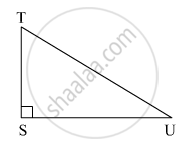Advertisement Remove all ads

# In Right Angled δ Tsu, Ts = 5, ∠ S = 90°, Su = 12 Then Find Sin T, Cos T, Tan T. Similarly Find Sin U, Cos U, Tan U. - Geometry

Sum

In right angled Δ TSU, TS = 5, ∠S = 90°, SU = 12 then find sin T, cos T, tan T. Similarly find sin U, cos U, tan U.Advertisement Remove all ads

#### SolutionIn right ∆TSU,
TU2 = SU2 + TS2              (Pythagoras theorem)
⇒ TU2 = 122 + 52 = 144 + 25 = 169
⇒ TU2 = 132
⇒ TU = 13
Now,

sin T = ["SU"]/["TU"]  = 12/13

cos T = ["TS"]/["TU"]  = 5/13

tan T = ["SU"]/["TS"]  = 12/5

Also,

sin U = ["TS"]/["TU"]  = 5/13

cos U = ["SU"]/["TU"]  = 12/13

tan U = ["TS"]/["SU"]  = 5/12

Is there an error in this question or solution?
Advertisement Remove all ads

#### APPEARS IN

Balbharati Mathematics 2 Geometry 9th Standard Maharashtra State Board
Chapter 8 Trigonometry
Problem Set 8 | Q 2 | Page 113
Advertisement Remove all ads
Advertisement Remove all ads
Share
Notifications

View all notifications

Forgot password?